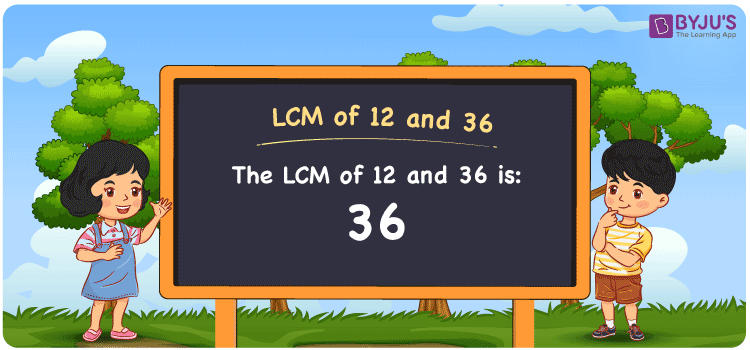Checkout JEE MAINS 2022 Question Paper Analysis : Checkout JEE MAINS 2022 Question Paper Analysis :

# LCM of 12 and 36

LCM of 12 and 36 is 36. Students will be able to learn how the least common multiple of 12 and 36 can be ontained from the common multiples. (12, 24, 36, 48, 60, 72, ….) and (36, 72, 108, 144, 180,….) are the multiples of 12 and 36. The methods which can be used to determine the LCM of two numbers are prime factorisation, by listing the multiples and division.

## What is LCM of 12 and 36?

The answer to this question is 36. The LCM of 12 and 36 using various methods is shown in this article for your reference. The LCM of two non-zero integers, 12 and 36, is the smallest positive integer 36 which is divisible by both 12 and 36 with no remainder.## How to Find LCM of 12 and 36?

LCM of 12 and 36 can be found using three methods:

• Prime Factorisation
• Division method
• Listing the multiples

### LCM of 12 and 36 Using Prime Factorisation Method

The prime factorisation of 12 and 36, respectively, is given by:

12 = 2 x 2 x 3 = 2² x 3¹

36 = 2 x 2 x 3 x 3 = 2² x 3²

LCM (12, 36) = 36

### LCM of 12 and 36 Using Division Method

We’ll divide the numbers (12, 36) by their prime factors to get the LCM of 12 and 36 using the division method (preferably common). The LCM of 12 and 36 is calculated by multiplying these divisors.

 2 12 36 2 6 18 3 3 9 3 1 3 x 1 1

No further division can be done.

Hence, LCM (12, 36) = 36

### LCM of 12 and 36 Using Listing the Multiples

To calculate the LCM of 12 and 36 by listing out the common multiples, list the multiples as shown below

 Multiples of 12 Multiples of 36 12 36 24 72 36 108 48 144 60 – 72 –

LCM (12, 36) = 36

## Video Lesson on Applications of LCM## LCM of 12 and 36 Solved Examples

Question: Determine the smallest number divisible by 12 and 36 exactly.

Solution:

We know that,

The smallest number exactly divisible by 12 and 36 is the LCM.

Multiples of 12 = 12, 24, 36, 48, 60,…..

Multiples of 36 = 36, 72, 108, 144

Hence, the LCM of 12 and 36 is 36.

## Frequently Asked Questions on LCM of 12 and 36

### In the numbers 2, 25, 5, 36, find the LCM of 12 and 36.

The smallest common multiple evenly divisible by 12 and 36 is the LCM value. The number 36 satisfies this condition.

### Find the GCF if the LCM of 12 and 36 is 36.

LCM x GCF = 12 x 36

Given

LCM of 12 and 36 = 36

36 x GCF = 432

GCF = 432/36 = 12

### Write the relation between GCF and LCM of 12 and 36.

The relation between GCF and LCM of 12 and 36 is

LCM x GCF = 12 x 36

LCM x GCF = 432